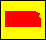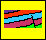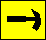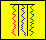Dakota Aquifer Program--Geologic Framework--Stochastic Modeling

## Initialization

The initialization phase of the program is the process when the observed data is put into the lattices includes the observed outcrop data, and subsurface data (from wells, as it becomes available). Also during this phase, we stochastically fill in some empty lattice nodes to decrease the sparseness of the observed field data. These nodes are filled according to the following algorithm:

We assign an initialization probability pk for each element k. Each probability pk is simply the relative frequency of occurrence of element k as observed in the field. Note that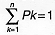where n is the total number of element types in the model. In the future work, we will iteratively adjust these probabilities pk to conform the output model to the observed data (see the final section for details).

Once the probabilities are calculated, a lattice node (i, j) is chosen at random. If the node is already filled with an element, we choose another (on the computer, this takes very little time, on average). If the node is empty we fill it with an element as follows: Generate a random number x between 0 and 1 (0 < x < 1). Partition the interval from 0 to 1 into n subintervals, where the mth subinterval has width pm. The starting point of each subinterval m is xm-1 and the ending point is xm, where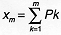(and x0=0). The relationship between the probabilities pm and the intervals [xm-1, xm] is illustrated below:Node (i, j) is filled with element m where m is such that xm-1 < x < xm. We continue filling the lattice unit 20% of the lattice nodes are filled. The model is now initialized and ready for the model generation phase.

Kansas Geological Survey, Dakota Aquifer Program
Updated July 5, 1996.
Scientific comments to P. Allen Macfarlane